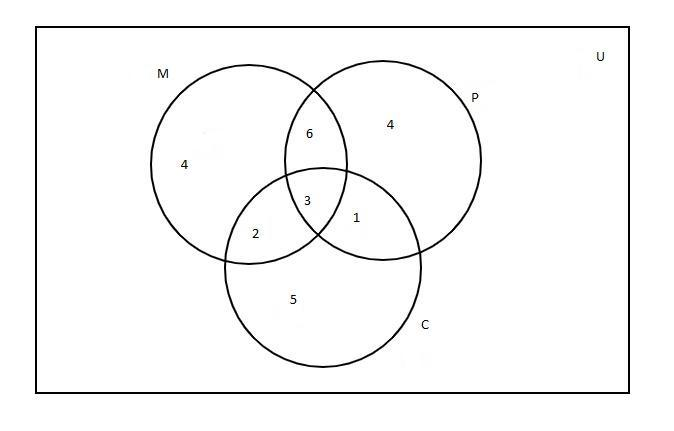QuestionAnswers

# In a survey of 25 students, it was found that 15 had taken mathematics, 12 had taken physics and 11 had taken chemistry, 5 had taken mathematics and chemistry, 9 had taken mathematics and physics, 4 had taken physics and chemistry and 3 had taken all the three subjects.Find the number of students that have taken none of the subjects.A. 0B. 2C. 3D. 5Verified
18.4K+ Views
Hint: In this question, first draw the Venn diagram with the given data it will give us a clear picture of what we have to find out. The number of students who had taken none of the subjects can be found by subtracting the number of students taken at least one subject from the total number of students in the survey. So, use this concept to reach the solution of the question.

Given,
Total number of students in the survey $n\left( U \right) = 25$

The set of students who have taken mathematics $n\left( M \right) = 15$
The set of students who have taken Physics $n\left( P \right) = 12$
The set of students who have taken chemistry $n\left( C \right) = 11$
The set of students taken mathematics and chemistry $n\left( {M \cap C} \right) = 5$
The set of students taken physics and chemistry $n\left( {P \cap C} \right) = 4$
The set of students taken mathematics and physics $n\left( {M \cap P} \right) = 9$
The set of students taken all the 3 subjects $n\left( {M \cap P \cap C} \right) = 3$
Here number of students taking at least one subject $= n\left( {M \cup P \cup C} \right)$

From the Venn diagram, we have$\Rightarrow n\left( {M \cup P \cup C} \right) = n\left( M \right) + n\left( P \right) + n\left( C \right) - n\left( {M \cap P} \right) - n\left( {P \cap C} \right) - n\left( {M \cap C} \right) + n\left( {M \cap P \cap C} \right) \\ \Rightarrow n\left( {M \cup P \cup C} \right) = 15 + 12 + 11 - 9 - 4 - 5 + 3 \\ \Rightarrow n\left( {M \cup P \cup C} \right) = 41 - 18 \\ \therefore n\left( {M \cup P \cup C} \right) = 23 \\$

So, the number of students taking none of the subjects $= n\left( U \right) - n\left( {M \cup P \cup C} \right) = 25 - 23 = 2$

Thus, the correct option is B i.e, 2

Note: The intersection of two sets $A$ and $B$, denoted by $A \cap B$, is the set containing all elements of $A$ that also belong to $B$ (or equivalently, all elements of $B$ that also belong to $A$). The union of two sets $A$ and $B$, denoted by $A \cup B$ is the set of all elements that are found in $A$ OR $B$ (or both)